## Online Education for RS Aggarwal Class 9 Solutions Chapter 13 Volume and Surface Area Ex 13B

These Solutions are part of Online Education RS Aggarwal Solutions Class 9. Here we have given RS Aggarwal Class 9 Solutions Chapter 13 Volume and Surface Area Ex 13B.

Other Exercises

Question 1.
Solution:
Radius of the base of a cylinder (r) = 5cm.
and height (h) = 21cm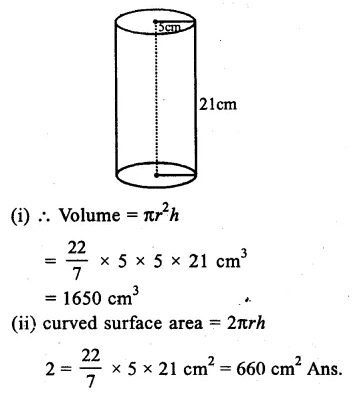Question 2.
Solution:
Diameter of the base of the cylinder = 28cm
Radius = $$\frac { 1 }{ 2 }$$ x 28 = 14 cm
Height (h) = 40cm.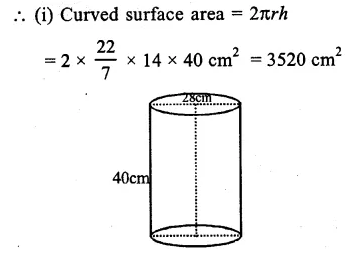Question 3.
Solution:
Radius of cylinder (r) = 10.5cm
Height (h) = 60cm.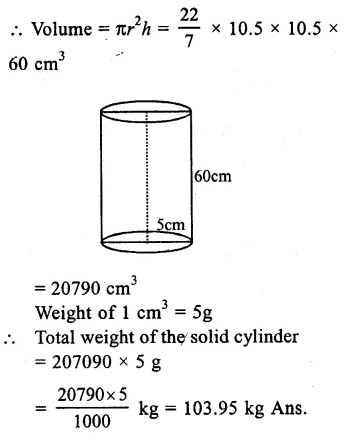Question 4.
Solution:
Diameter of cylinder = 20cm
Radius (r) = $$\frac { 20 }{ 2 }$$ = 10cm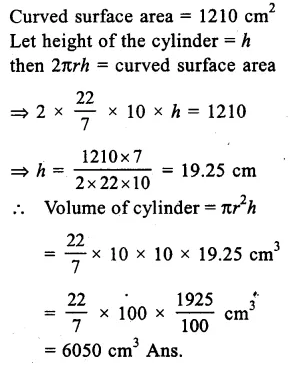Question 5.
Solution:
Curved surface area of cylinder = 4400 cm²
Circumference of its base = 110 cm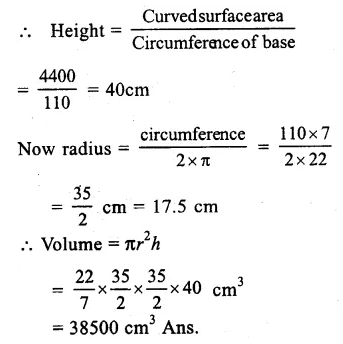Question 6.
Solution:
The ratio of the radius and height of a cylinder = 2:3
Volume =1617 cm³
and height = 3x.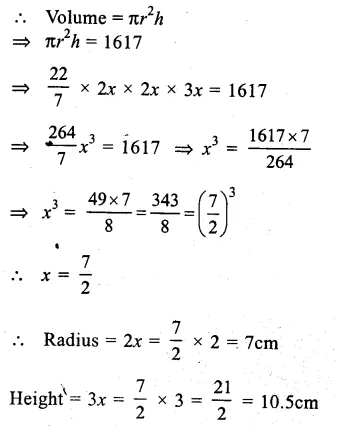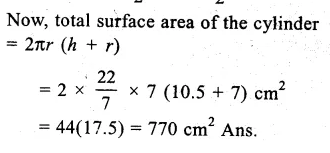Question 7.
Solution:
Total surface area of the cylinder = 462 cm²
Curved surface area = $$\frac { 1 }{ 3 }$$ x 462 = 154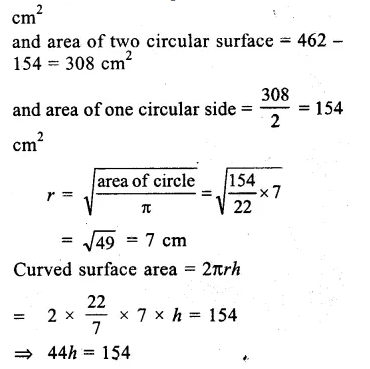Question 8.
Solution:
Total surface area of solid
cylinder = 231 cm²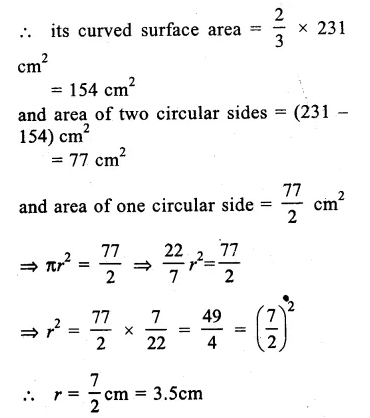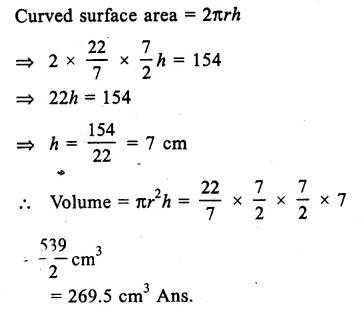Question 9.
Solution:
Sum of radius and height = 37m.
and total surface area = 1628 m²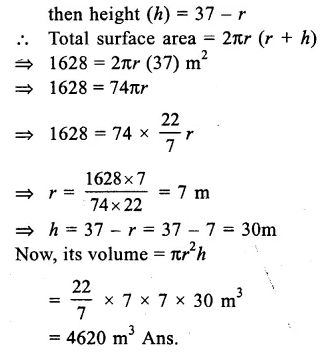Question 10.
Solution:
Total surface area = 616 cm²
Curved surface area = $$\frac { 616X1 }{ 2 }$$ = 308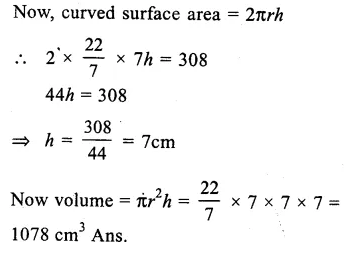Question 11.
Solution:
Volume of gold = 1 cm³
diameter of wire = 0.1 mnQuestion 12.
Solution:
Ratio in the radii of two cylinders = 2:3
and ratio in the heights = 5:3
If r1 and r2 and the radii and h1 and h2 are the heights, then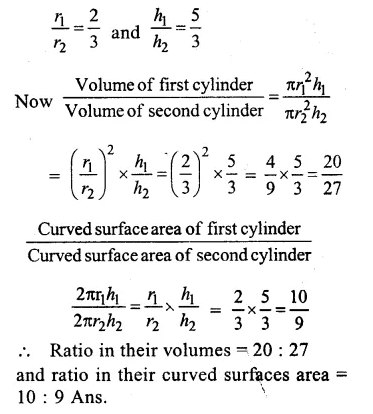Question 13.
Solution:
Side of square = 12cm
and height = 17.5cmQuestion 14.
Solution:
Diameter of cylindrical bucket = 28cm
Radius (r) = $$\frac { 28 }{ 8 }$$ = 14cm
Height (h) = 72cm.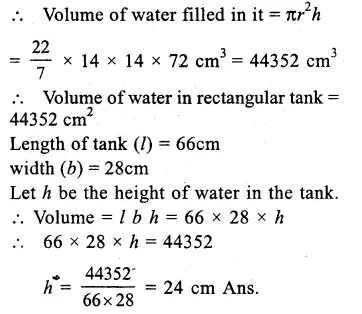Question 15.
Solution:
Length of pipe (l) = 1m = 100cm
diameter of pipe = 3cm.
Inner radius = $$\frac { 3 }{ 2 }$$ cmQuestion 16.
Solution:
Internal diameter of cylindrical tube = 10.4 cm
Radius (r) = $$\frac { 10.4 }{ 2 }$$ = 5.2cm.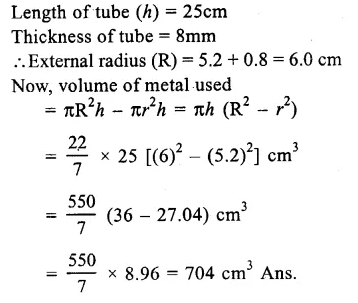Question 17.
Solution:
Length of barrel (h) = 7cm
Diameter = 5mm.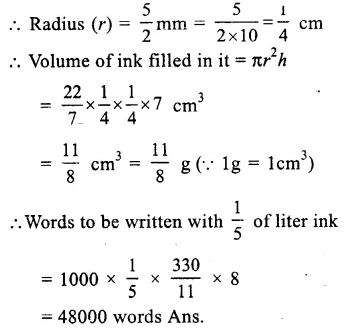Question 18.
Solution:
Diameter of pencil = 7mm
.’. Radius (R) = $$\frac { 7 }{ 2 }$$ mm = $$\frac { 7 }{ 20 }$$ cm.
and diameter of graphite in it = 1mm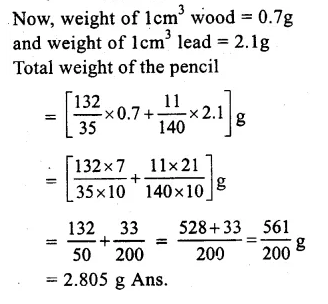Hope given RS Aggarwal Class 9 Solutions Chapter 13 Volume and Surface Area Ex 13B are helpful to complete your math homework.

If you have any doubts, please comment below. Learn Insta try to provide online math tutoring for you.